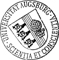## Numerical Simulation of the Motion and Deformation of Red Blood Cells and Vesicles in Microfluidic Flows

• We study the mathematical modeling and numerical simulation of the motion and deformation of red blood cells (RBC) and vesicles subject to an external incompressible flow in a microchannel. RBC and vesicles are viscoelastic bodies consisting of a deformable elastic membrane enclosing an incompressible fluid. We provide an extension of the Finite Element Immersed Boundary Method based on a model for the membrane that additionally accounts for bending energy and also consider inflow/outflow conditions for the external fluid flow. The stability analysis requires both the approximation of the membrane by cubic splines (instead of linear splines without bending energy) and an upper bound on the inflow velocity. In the fully discrete case, the resulting CFL-type condition on the time step size is also more restrictive. We perform numerical simulations for various scenarios including the tank treading motion of vesicles in microchannels, the behavior of 'healthy' and 'sick' RBC which differWe study the mathematical modeling and numerical simulation of the motion and deformation of red blood cells (RBC) and vesicles subject to an external incompressible flow in a microchannel. RBC and vesicles are viscoelastic bodies consisting of a deformable elastic membrane enclosing an incompressible fluid. We provide an extension of the Finite Element Immersed Boundary Method based on a model for the membrane that additionally accounts for bending energy and also consider inflow/outflow conditions for the external fluid flow. The stability analysis requires both the approximation of the membrane by cubic splines (instead of linear splines without bending energy) and an upper bound on the inflow velocity. In the fully discrete case, the resulting CFL-type condition on the time step size is also more restrictive. We perform numerical simulations for various scenarios including the tank treading motion of vesicles in microchannels, the behavior of 'healthy' and 'sick' RBC which differ by their stiffness, and the motion of RBC through thin capillaries. The simulation results are in very good agreement with experimentally available data.• Dokument_1.pdfAuthor: Thomas FrankeGND, Ronald H. W. HoppeGND, Christopher LinsenmannGND, Lothar Schmid, Carina Willbold, Achim WixforthORCiDGND urn:nbn:de:bvb:384-opus4-5359 https://opus.bibliothek.uni-augsburg.de/opus4/677 Preprints des Instituts für Mathematik der Universität Augsburg (2010-09) Preprint English Universität Augsburg University of Houston 2010/06/17 finite element immersed boundary method; microfluidic flows; red blood cells; vesicles Strömungsmechanik; Mathematische Modellierung; Numerische Strömungssimulation; Finite-Elemente-Methode; Retikulozyt; Mikrofluidik Mathematisch-Naturwissenschaftlich-Technische Fakultät Mathematisch-Naturwissenschaftlich-Technische Fakultät / Institut für Mathematik Mathematisch-Naturwissenschaftlich-Technische Fakultät / Institut für Physik Mathematisch-Naturwissenschaftlich-Technische Fakultät / Institut für Mathematik / Lehrstuhl für Numerische Mathematik Mathematisch-Naturwissenschaftlich-Technische Fakultät / Institut für Physik / Lehrstuhl für Experimentalphysik I 5 Naturwissenschaften und Mathematik / 51 Mathematik / 510 MathematikDeutsches Urheberrecht mit Print on Demand